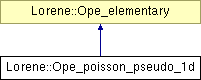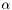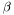# Lorene::Ope_poisson_pseudo_1d Class Reference

Class for the operator of the Poisson equation in pseudo 1d. More...

#include <ope_elementary.h>

Inheritance diagram for Lorene::Ope_poisson_pseudo_1d:List of all members.

## Public Member Functions

Ope_poisson_pseudo_1d (int nbr, int baser, double alf, double bet, int lq)
Standard constructor.
Ope_poisson_pseudo_1d (const Ope_poisson_pseudo_1d &)
Constructor by copy.
virtual ~Ope_poisson_pseudo_1d ()
Destructor.
int get_lquant ()
Returns the quantum number l.
virtual Tbl get_solp (const Tbl &so) const
Computes the particular solution, given the source so .
virtual Tbl get_solh () const
Computes the homogeneous solutions(s).
virtual void inc_l_quant ()
Increases the quatum number l by one unit.
virtual void dec_l_quant ()
Decreases the quatum number l by one unit.
double val_sh_one_minus () const
Returns the value of the first homogeneous solution at the inner boundary.
double val_sh_one_plus () const
Returns the value of the first homogeneous solution at the outer boundary.
double der_sh_one_minus () const
Returns the value of the derivative of the first homogeneous solution at the inner boundary.
double der_sh_one_plus () const
Returns the value of the derivative of the first homogeneous solution at the outer boundary.
double val_sh_two_minus () const
Returns the value of the second homogeneous solution at the inner boundary.
double val_sh_two_plus () const
Returns the value of the second homogeneous solution at the outer boundary.
double der_sh_two_minus () const
Returns the value of the derivative of the second homogeneous solution at the inner boundary.
double der_sh_two_plus () const
Returns the value of the derivative of the second homogeneous solution at the outer boundary.
double val_sp_minus () const
Returns the value of the particular solution at the inner boundary.
double val_sp_plus () const
Returns the value of the particular solution at the outer boundary.
double der_sp_minus () const
Returns the value of the derivative particular solution at the inner boundary.
double der_sp_plus () const
Returns the value of the derivative particular solution at the outer boundary.
double get_alpha () const
Returns alpha .
double get_beta () const
Returns beta}.
int get_base_r () const
Returns base_r}.
Matrice get_ope_mat ()
Returns the matrix representation.
Matrice get_ope_cl ()
Returns the banded matrix representation.
Matrice get_non_dege ()
Returns the non degenerate matrix representation.

## Protected Attributes

int l_quant
quantum number
int nr
int base_r
double alpha
Parameterof the associated mapping.
double beta
Parameterof the associated mapping.
Matriceope_mat
Pointer on the matrix representation of the operator.
Matriceope_cl
Pointer on the banded-matrix of the operator.
Matricenon_dege
Pointer on the non-degenerated matrix of the operator.
double s_one_plus
Value of the first homogeneous solution at the outer boundary.
double s_one_minus
Value of the first homogeneous solution at the inner boundary.
double ds_one_plus
Value of the derivative of the first homogeneous solution at the outer boundary.
double ds_one_minus
Value of the derivative of the first homogeneous solution at the inner boundary.
double s_two_plus
Value of the second homogeneous solution at the outer boundary.
double s_two_minus
Value of the second homogeneous solution at the inner boundary.
double ds_two_plus
Value of the derivative of the second homogeneous solution at the outer boundary.
double ds_two_minus
Value of the derivative of the second homogeneous solution at the inner boundary.
double sp_minus
Value of the particular solution at the inner boundary.
double sp_plus
Value of the particular solution at the outer boundary.
double dsp_minus
Value of the derivative of the particular solution at the inner boundary.
double dsp_plus
Value of the derivative of the particular solution at the outer boundary.

## Private Member Functions

virtual void do_ope_mat () const
Computes the matrix of the operator.
virtual void do_ope_cl () const
Computes the banded-matrix of the operator.
virtual void do_non_dege () const
Computes the non-degenerated matrix of the operator.

## Detailed Description

Class for the operator of the Poisson equation in pseudo 1d.

Definition at line 859 of file ope_elementary.h.

## Constructor & Destructor Documentation

 Lorene::Ope_poisson_pseudo_1d::Ope_poisson_pseudo_1d ( int nbr, int baser, double alf, double bet, int lq )

Standard constructor.

Parameters:
 nbr [input] number of radial points. baser [input] radial basis of decomposition. alf [input] parameterof the mapping. bet [input] parameterof the mapping. lq [input] quantum number l .

Definition at line 50 of file ope_poisson_pseudo_1d.C.

 Lorene::Ope_poisson_pseudo_1d::Ope_poisson_pseudo_1d ( const Ope_poisson_pseudo_1d & so )

Constructor by copy.

Definition at line 56 of file ope_poisson_pseudo_1d.C.

 Lorene::Ope_poisson_pseudo_1d::~Ope_poisson_pseudo_1d ( )  [virtual]

Destructor.

Definition at line 62 of file ope_poisson_pseudo_1d.C.

## Member Function Documentation

 void Lorene::Ope_poisson_pseudo_1d::dec_l_quant ( )  [virtual]

Decreases the quatum number l by one unit.

Definition at line 70 of file ope_poisson_pseudo_1d.C.

 double Lorene::Ope_elementary::der_sh_one_minus ( ) const [inline, inherited]

Returns the value of the derivative of the first homogeneous solution at the inner boundary.

Definition at line 211 of file ope_elementary.h.

References Lorene::Ope_elementary::ds_one_minus.

 double Lorene::Ope_elementary::der_sh_one_plus ( ) const [inline, inherited]

Returns the value of the derivative of the first homogeneous solution at the outer boundary.

Definition at line 217 of file ope_elementary.h.

References Lorene::Ope_elementary::ds_one_plus.

 double Lorene::Ope_elementary::der_sh_two_minus ( ) const [inline, inherited]

Returns the value of the derivative of the second homogeneous solution at the inner boundary.

Definition at line 234 of file ope_elementary.h.

References Lorene::Ope_elementary::ds_two_minus.

 double Lorene::Ope_elementary::der_sh_two_plus ( ) const [inline, inherited]

Returns the value of the derivative of the second homogeneous solution at the outer boundary.

Definition at line 240 of file ope_elementary.h.

References Lorene::Ope_elementary::ds_two_plus.

 double Lorene::Ope_elementary::der_sp_minus ( ) const [inline, inherited]

Returns the value of the derivative particular solution at the inner boundary.

Definition at line 254 of file ope_elementary.h.

References Lorene::Ope_elementary::dsp_minus.

 double Lorene::Ope_elementary::der_sp_plus ( ) const [inline, inherited]

Returns the value of the derivative particular solution at the outer boundary.

Definition at line 259 of file ope_elementary.h.

References Lorene::Ope_elementary::dsp_plus.

 void Lorene::Ope_poisson_pseudo_1d::do_non_dege ( ) const [private, virtual]

Computes the non-degenerated matrix of the operator.

Implements Lorene::Ope_elementary.

Definition at line 145 of file ope_poisson_pseudo_1d_non_dege.C.

 void Lorene::Ope_poisson_pseudo_1d::do_ope_cl ( ) const [private, virtual]

Computes the banded-matrix of the operator.

Implements Lorene::Ope_elementary.

Definition at line 131 of file ope_poisson_pseudo_1d_cl.C.

 void Lorene::Ope_poisson_pseudo_1d::do_ope_mat ( ) const [private, virtual]

Computes the matrix of the operator.

Implements Lorene::Ope_elementary.

Definition at line 195 of file ope_poisson_pseudo_1d_mat.C.

 double Lorene::Ope_elementary::get_alpha ( ) const [inline, inherited]

Returns alpha .

Definition at line 262 of file ope_elementary.h.

References Lorene::Ope_elementary::alpha.

 int Lorene::Ope_elementary::get_base_r ( ) const [inline, inherited]

Returns base_r}.

Definition at line 268 of file ope_elementary.h.

References Lorene::Ope_elementary::base_r.

 double Lorene::Ope_elementary::get_beta ( ) const [inline, inherited]

Returns beta}.

Definition at line 265 of file ope_elementary.h.

References Lorene::Ope_elementary::beta.

 int Lorene::Ope_poisson_pseudo_1d::get_lquant ( )  [inline]

Returns the quantum number l.

Definition at line 879 of file ope_elementary.h.

References l_quant.

 Matrice Lorene::Ope_elementary::get_non_dege ( )  [inline, inherited]

Returns the non degenerate matrix representation.

Definition at line 285 of file ope_elementary.h.

 Matrice Lorene::Ope_elementary::get_ope_cl ( )  [inline, inherited]

Returns the banded matrix representation.

Definition at line 278 of file ope_elementary.h.

 Matrice Lorene::Ope_elementary::get_ope_mat ( )  [inline, inherited]

Returns the matrix representation.

Definition at line 271 of file ope_elementary.h.

 Tbl Lorene::Ope_poisson_pseudo_1d::get_solh ( ) const [virtual]

Computes the homogeneous solutions(s).

Implements Lorene::Ope_elementary.

Definition at line 183 of file ope_poisson_pseudo_1d_solh.C.

 Tbl Lorene::Ope_poisson_pseudo_1d::get_solp ( const Tbl & so ) const [virtual]

Computes the particular solution, given the source so .

Implements Lorene::Ope_elementary.

Definition at line 285 of file ope_poisson_pseudo_1d_solp.C.

 void Lorene::Ope_poisson_pseudo_1d::inc_l_quant ( )  [virtual]

Increases the quatum number l by one unit.

Implements Lorene::Ope_elementary.

Definition at line 64 of file ope_poisson_pseudo_1d.C.

 double Lorene::Ope_elementary::val_sh_one_minus ( ) const [inline, inherited]

Returns the value of the first homogeneous solution at the inner boundary.

Definition at line 200 of file ope_elementary.h.

References Lorene::Ope_elementary::s_one_minus.

 double Lorene::Ope_elementary::val_sh_one_plus ( ) const [inline, inherited]

Returns the value of the first homogeneous solution at the outer boundary.

Definition at line 205 of file ope_elementary.h.

References Lorene::Ope_elementary::s_one_plus.

 double Lorene::Ope_elementary::val_sh_two_minus ( ) const [inline, inherited]

Returns the value of the second homogeneous solution at the inner boundary.

Definition at line 223 of file ope_elementary.h.

References Lorene::Ope_elementary::s_two_minus.

 double Lorene::Ope_elementary::val_sh_two_plus ( ) const [inline, inherited]

Returns the value of the second homogeneous solution at the outer boundary.

Definition at line 228 of file ope_elementary.h.

References Lorene::Ope_elementary::s_two_plus.

 double Lorene::Ope_elementary::val_sp_minus ( ) const [inline, inherited]

Returns the value of the particular solution at the inner boundary.

Definition at line 245 of file ope_elementary.h.

References Lorene::Ope_elementary::sp_minus.

 double Lorene::Ope_elementary::val_sp_plus ( ) const [inline, inherited]

Returns the value of the particular solution at the outer boundary.

Definition at line 249 of file ope_elementary.h.

References Lorene::Ope_elementary::sp_plus.

## Member Data Documentation

 double Lorene::Ope_elementary::alpha [protected, inherited]

Parameterof the associated mapping.

Definition at line 108 of file ope_elementary.h.

 int Lorene::Ope_elementary::base_r [protected, inherited]

Definition at line 107 of file ope_elementary.h.

 double Lorene::Ope_elementary::beta [protected, inherited]

Parameterof the associated mapping.

Definition at line 109 of file ope_elementary.h.

 double Lorene::Ope_elementary::ds_one_minus [mutable, protected, inherited]

Value of the derivative of the first homogeneous solution at the inner boundary.

Definition at line 141 of file ope_elementary.h.

 double Lorene::Ope_elementary::ds_one_plus [mutable, protected, inherited]

Value of the derivative of the first homogeneous solution at the outer boundary.

Definition at line 136 of file ope_elementary.h.

 double Lorene::Ope_elementary::ds_two_minus [mutable, protected, inherited]

Value of the derivative of the second homogeneous solution at the inner boundary.

Definition at line 160 of file ope_elementary.h.

 double Lorene::Ope_elementary::ds_two_plus [mutable, protected, inherited]

Value of the derivative of the second homogeneous solution at the outer boundary.

Definition at line 155 of file ope_elementary.h.

 double Lorene::Ope_elementary::dsp_minus [mutable, protected, inherited]

Value of the derivative of the particular solution at the inner boundary.

Definition at line 173 of file ope_elementary.h.

 double Lorene::Ope_elementary::dsp_plus [mutable, protected, inherited]

Value of the derivative of the particular solution at the outer boundary.

Definition at line 177 of file ope_elementary.h.

 int Lorene::Ope_poisson_pseudo_1d::l_quant [protected]

quantum number

Definition at line 862 of file ope_elementary.h.

 Matrice* Lorene::Ope_elementary::non_dege [mutable, protected, inherited]

Pointer on the non-degenerated matrix of the operator.

Definition at line 122 of file ope_elementary.h.

 int Lorene::Ope_elementary::nr [protected, inherited]

Definition at line 106 of file ope_elementary.h.

 Matrice* Lorene::Ope_elementary::ope_cl [mutable, protected, inherited]

Pointer on the banded-matrix of the operator.

Definition at line 118 of file ope_elementary.h.

 Matrice* Lorene::Ope_elementary::ope_mat [mutable, protected, inherited]

Pointer on the matrix representation of the operator.

Definition at line 114 of file ope_elementary.h.

 double Lorene::Ope_elementary::s_one_minus [mutable, protected, inherited]

Value of the first homogeneous solution at the inner boundary.

Definition at line 131 of file ope_elementary.h.

 double Lorene::Ope_elementary::s_one_plus [mutable, protected, inherited]

Value of the first homogeneous solution at the outer boundary.

Definition at line 127 of file ope_elementary.h.

 double Lorene::Ope_elementary::s_two_minus [mutable, protected, inherited]

Value of the second homogeneous solution at the inner boundary.

Definition at line 150 of file ope_elementary.h.

 double Lorene::Ope_elementary::s_two_plus [mutable, protected, inherited]

Value of the second homogeneous solution at the outer boundary.

Definition at line 146 of file ope_elementary.h.

 double Lorene::Ope_elementary::sp_minus [mutable, protected, inherited]

Value of the particular solution at the inner boundary.

Definition at line 165 of file ope_elementary.h.

 double Lorene::Ope_elementary::sp_plus [mutable, protected, inherited]

Value of the particular solution at the outer boundary.

Definition at line 169 of file ope_elementary.h.

The documentation for this class was generated from the following files:

Generated on 7 Dec 2019 for LORENE by1.6.1